IF YOU CAN SEE THIS go to /sfa/Nuprl/Shared/Xindentation_hack_doc.html

### Quotient Types (of equivalence classes)

u,v:A//E(u;v) is the quotient of type A by equivalence relation E(u;v) for u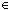A, vA. The members are equivalence classes. See Equality.

However, in Nuprl we do not employ explicit coercions from members of A to equivalence classes under E(u;v). Rather, we just use the same notations for both members and equivalence classes containing them, which entails that we must sometimes specify which is meant (though often we can simply elide the type from display).

For example, Thm* 2 = 4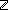mod 2, where

Defmod k == x,y://(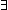m:x-y = m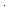k).

The semantics of quotient types is, for xA, yA,
x = yu,v:A//E(u;v) when E(x;y).

The Intensional Type Equality between quotients is not strong in the equivalence relation. The types u,v:A//E(u;v) and u,v:B//R(u;v) are intensionally equal when A and B are, and also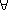u,v:AE(u;v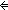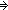R(u;v).